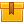# Level Measurement (AFM04)

## Prerequisites

This lesson is designed for participants familiar with basic mathematical operations including algebra.

## Description

This lesson describes the fundamentals of level measurement and the sensors employed. Applications for both direct and indirect level measurement are covered including float-type devices, hydrostatic head and differential pressure measurements, as well as electrical, ultrasonic, and radiation instruments.

## Objectives

Recognize the importance of measuring and controlling level. Describe what an interface is and list some of the types of interfaces that may be measured for level indication. List common measurement units of level. Define direct and indirect level measurement and some types and applications of these methods. Define continuous level and point level measurement. Describe how sight glasses operate to measure liquid level. Describe how dipsticks, weighted lines, and float-type instruments can be used to gage level. Define hydrostatic head pressure and explain how it can be used to measure the height of liquid. Calculate the height of the liquid in inches with a head pressure and specific gravity. Describe configurations using hydrostatic head to measure level in open tanks. Describe how differential pressure can be used to measure level in closed-tank applications using a dry or wet leg. Explain how level can be measured using electrical capacitance or resistance. Describe two ways the level of granular solids and powders can be measured. Describe some non-invasive level measurement methods that use ultrasonic and radiation detectors. Describe the basic operation of one type of fiber optic level measurement instrument for point level measurement.

## ContentThis lesson describes the fundamentals of level measurement and the
sensors employed. Applications for both direct and indirect level
measurement are covered including float-type devices, hydrostatic head
and differential pressure measurements, as well as electrical,
•Level Measurement